## Section 5.3  ELECTROMAGNETIC RELATIONSGaussian CGS SI Units and conversions: Charge 2.997 92 ´ 109 esu = 1 C = 1 A s Potential (1/299.792) statvolt = (1/299.792) erg/esu = 1 V = 1 J C–1 Magnetic field 104 gauss = 104 dyne/esu = 1 T = 1 N A–1 m–1 Electron charge e = 4.803 204 ´ 10–10 esu = 1.602 176 ´ 10–19 C Lorentz force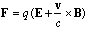Maxwell equations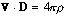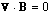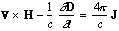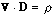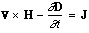Linear media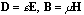Permittivity of free space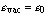Permeability of free space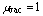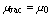Fields from potentials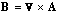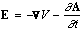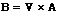Static potentials (coulomb gauge)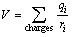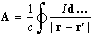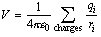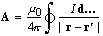Relativistic transformations (v is the velocity of primed system as seen in un- primed system)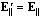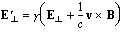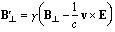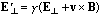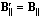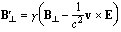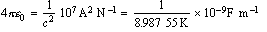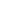Impedances (SI units)

r = resistivity at room temperature in 10–8 W m:

~ 1.7 for Cu                         ~ 5.5 for W

~ 2.4 for Au                         ~ 73 for SS 304

~ 2.8 for Al                          ~ 100 for Nichrome

(Al alloys may have
double this value.)

For alternating currents, instantaneous current I, voltage V, angular frequency w:.

Impedance of self-inductance L:  Z = jwL .

Impedance of capacitance C:  Z = 1/jwC .

Impedance of free space: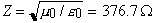.

High-frequency surface impedance of a good conductor: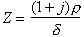, where d = effective skin depth ;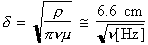for Cu .

Capacitance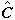and inductance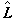per unit length (SI units)

Flat rectangular plates of width w, separated by d << w with linear medium (e, m) between: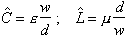;= 2 to 6 for plastics; 4 to 8 for porcelain, glasses;Coaxial cable of inner radius r1, outer radius r2: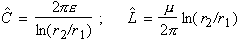.

Transmission lines (no loss):

Impedance: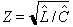.

Velocity: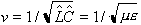.

Motion of charged particles in a uniform, static magnetic field

The path of motion of a charged particle of momentum p is a helix of constant radius R and constant pitch angle l, with the axis of the helix along B:,

where the charge q is in units of the electronic charge.  The angular velocity about the axis of the helix is,

where E is the energy of the particle.

This section was adapted, with permission, from the 1999 web edition of the Review of Particle Physics (http://pdg. lbl.gov). See J. D. Jackson, Classical Electrodynamics, 2d ed. (John Wiley & Sons, New York, 1975) for more formulas and details. A PDF version of this table is also available.